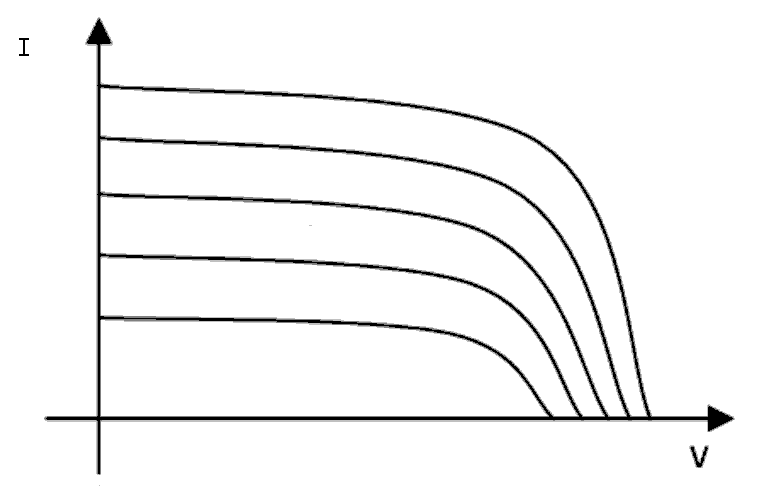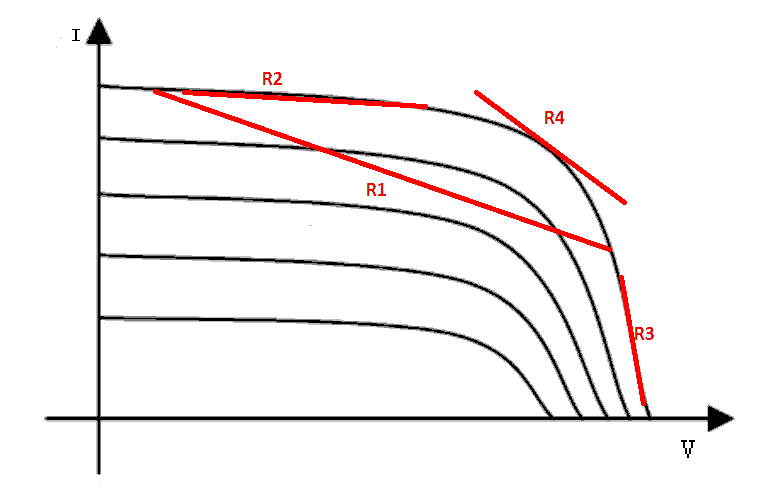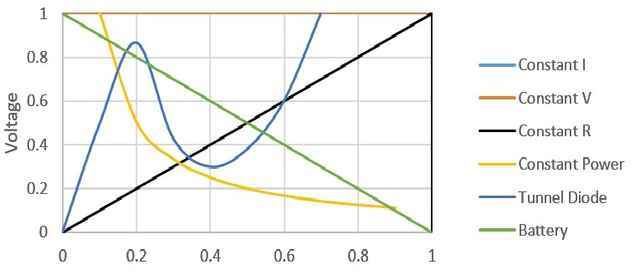# Why Ohm’s Law Is Not a Law

[Total: 6    Average: 4.2/5]

At first I wanted this title to say “Ohm’s law is not a Law.”  But someone else used that phrase in a recent PF thread, and a storm of protest followed.   We are talking about the relationship between Voltage between two points in a circuit and the current between those same two points.

##R=V/I##, or ##V=IR##, or ##I=V/R##

I won’t explicitly talk about inductance or capacitance, or AC impedances, although all of those could substitute for ##R## in the following discussion.

### Semantics

Newton’s Second Law can be derived from fundamental symmetries in nature.  But Ohm’s law is not derived, it was empirically discovered by Georg Ohm.  He found materials that have a linear relationship of voltage to current within a specified range are called “ohmic.”  Many useful materials and useful electric devices are non-ohmic.

Beginners often believe that there is great significance in the use of words like “law” or “theory” In physics.  Not so.  Often it is just an accident of history or a phrase that slides easily off the tongue.  It could have been called Ohm’s rule or Ohm’s observation, but it wasn’t.

### Underlying Assumptions and Limitations

Really there is only one assumption behind Ohm’s law; linearity.   Not in the mathematical sense, but rather that a graph of voltage versus current shows an approximately straight line in a given range.

There are always limits to the range even though they may not be explicitly mentioned.  For example, at high voltages breakdown and arcing can occur.  At high currents, things tend to melt.    In the old days, we said that real-world resistance can not be zero. But now we know that superconductors are an exception to that rule.  ##R=0## is OK for superconductors.

Students often forget that limits exist.   A frequent (and annoying) student question is, “So if ##I=V/R##, what happens when ##R=0##.  Ha ha, LOL.”  They think that disproves the “law” and thus diminishes the credibility of science in general.  Their logic is false.

### Ohm’s law Has Several Forms

##I=V/R## may be the most familiar form of Ohm’s law, but it is far from the only one.

• In AC analysis, we use ##\bar V=\bar I\bar Z##, where ##\bar V##, ##\bar I## and ##\bar Z## are all complex vectors. ##\bar Z## is the complex impedance that can describe combinations of resistance, inductance and capacitance, without differential equations.  See PF Insights AC Power Analysis, Part 1 Basics.
• ##\overrightarrow E=\rho \overrightarrow J## is the continuous form of Ohm’s law. where ##\overrightarrow E## is the electric field vector with units of volts per meter (analogous to ##V## of Ohm’s law which has units of volts), ##\overrightarrow J## is the current density vector with units of amperes per unit area (analogous to ##I## of Ohm’s law which has units of amperes), and  ##\rho## is the resistivity with units of ohm·meters.   Use this form to model currents in 3-dimensional space.
• If an external ##B##-field is present and the conductor is not at rest but moving at velocity ##v##, then we use: ##(\overrightarrow E + v \times \overrightarrow B)=\rho \overrightarrow J##
• There are also variants of Ohm’s law for 2 D sheets, for magnetic circuits (Hopkinson’s Law), Frick’s Law for diffusion dominated cases, and for semiconductors.

Someone on PF once said that one form was the only “true” Ohm’s law.  I disagree.  All the forms are useful in different contexts.   We can honor Georg Ohm by using his name to cover all the variants, even if he didn’t personally invent all of them.

### Real Life Example, A Solar Panel

A solar panel is a real life device.  Below, we see a family of curves showing the Voltage V versus current I relationship of a solar panel.  Each curve represents a different value of solar intensity.  As you can see, the curves range from nearly an ideal voltage source (vertical), to a nearly ideal current source (horizontal), to everything in between.We can draw straight line segments to define the average resistance over a particular range, like R1, R2 or R3.  As you can see, the panel ranges from nearly an ideal voltage source, to a nearly ideal current source, to everything in between.  R4 shows a resistance defined by the tangent to the curve at one particular point.  I call R4 the resistance linearized about a point on the curve.We could use these resistances, plus a Thevanin’s Equivalent voltage ##V_{thev}##  to model the solar panel in a circuit to be solved using Ohm’s law. (##V_{thev}##  is  the place where the straight line intercepts the ##V## axis or where ##I=0##).

### Arbitrary Numbers of Arbitrary Electric Devices

When we go beyond a simple resistor made of some material with a linear V-I relationship, we find that very many real-world devices are non-linear.  For example, the constant power device (yellow) and the tunnel diode device (blue) curves seen below.   There are no physical constraints on the shape of a V-I relationship other than that both V and I must be finite.  Even a multi-valued curve that loops back on itself violates no physical law.Students in elementary circuits courses often learn 0nly about the constant R (i.e. the resistor) element, plus L and C.  Other kinds of nonlinear circuit devices may not even be mentioned.

At first glance, you might say that Ohm’s law doesn’t apply to nonlinear devices, but that’s not true. Suppose we had a circuit containing a solar panel, a constant power and a tunnel diode.  A student using paper and pencil could not be asked to solve such a circuit, so courses that limit themselves to paper and pencil methods do not cover nonlinear elements.  But using computers, there is a relatively simple method:

1. Guess an initial V and I point along the curve for each device.
2. Find the linearized resistance (analogous to R4) for each device at the V and I guess point.
3. Solve the linearized circuit using Ohm’s law, calculating new values for each V and I.
4. Use the calculated V and I as the new guess and return to step 2.

An iteration like that is very easy to perform with a computer.  It isn’t guaranteed to succeed, but when it does succeed, after several passes through steps 2 and 3, it will calculate values for V and I that simultaneously satisfy the relationships of all linear and nonlinear elements in the circuit.    It is routine in power grid analysis to solve circuits with a million or more diverse nonlinear elements.  Thus, even when modeling devices that don’t seem to obey Ohm’s law, that we can make productive use of Ohm’s law nevertheless.  I’ll say it again using stronger words. Ohm’s law cannot be violated in real life; rather it can be adapted to nearly all real life situations in electric circuits.

Ohm’s law is not always a complete description of electrical devices, but Ohm’s law is almost always a useful tool.   Students are advised to learn to think that way.  Physicists and engineers seek usefulness and try to leave truth to philosophers.

### Electricity Study Levels

One can study and explain electricity at (at least) 5 levels.

In this article, my focus was circuit analysis.   One of the standard assumptions for circuit analysis is that Kirchoffs laws apply instantaneously to the entire circuit.  That makes ##V##  ##I## and ##R## co-equal partners.  None of the three can be said to be cause and the other effect.  All three apply simultaneously.

Students dissatisfied with Circuit Analysis sometimes yearn for physical explanations and invent false narratives about what happens first and what comes next.  If you are such a student, I urge you to study Circuit Analysis first, then follow-up with the other levels.  If that describes you, I advise that the next step is to abandon circuit analysis, Ohm’s law, Kirchoff’s laws, and to learn Maxwell’s equation as the next deeper step.  Fields, not electron motion are the key to the next deeper step.

Thanks to PF regular @Jim Hardy for his assistance.

41 replies
1.Greg Bernhardt says:

A question from Reddit

The ideal gas law doesn't apply for real gases. Is the ideal gas law then not a law?

2.eltodesukane says:

Semantics..Just like Moore's law (which is not a fundamental law of the universe), or evolution theory (which so many have told me is "just a theory").

3.Averagesupernova says:

I have actually thought about this a number of times.  I look at it from the perspective that ohms is simply a ratio.  Sometimes the ratio holds true across a wide range of voltages and currents and with other materials not so much.  We can nitpick about this from now until eternity I suppose.

4.LvW says:

…and we can think about the meaning of the form: V=I*R.

We are using this form to find the "voltage drop" caused by a current I that goes through the resitor R.

However, is it – physikally spoken – correct to say that the current I is producing a voltage V across the resistor R ?

(Because an electrical field within the resistive body is a precondition for a current I, is it not?)

5.Dr. Courtney says:

There are important distinctions between truly general laws and useful summaries that are technically approximations at best and in some ways more definitions of tautologies.  Coulomb's law is a true law of Physics.Ohm's law is more of a definition of Ohmic materials.  Ohmic materials obey Ohm's law.  That's like saying "It works where it works" without having real predictive power regarding for what materials it will and won't work before the experiment is performed.The ideal gas law is an approximation in a limiting case.  In that sense, it is a true law of Physics, at least as much of Galileo's law of falling bodies.

6.vanhees71 says:

Well, it's really semantics. I'd not say that Coulomb's law is "true law of physics" although I prefer the phrase "fundamental law". All physics laws are "true" in the sense that they are well tested by observations and in most cases have a restricted range of validity.

That said, I think that Coulomb's Law is, as Ohm's Law, a "derived law" from the fundamental laws of (quantum) electrodynamics. Coulomb's Law is the electrostatic field of a radially symmetric static charge distribution and of course derivable from Maxwell's equations. Ohm's Law is derived from linear-response theory of (quantum) electrodynamics. The electric conductivity is a bona-fide transport coefficient, definable in terms of the Kubo formula. The corresponding correlation function is the em. current-current correlator etc.

7.Averagesupernova says:

…and we can think about the meaning of the form: V=I*R.

We are using this form to find the "voltage drop" caused by a current I that goes through the resistor R.

However, is it – physically spoken – correct to say that the current I is producing a voltage V across the resistor R ?

(Because an electrical field within the resistive body is a precondition for a current I, is it not?)

We can determine the speed, distance, or rate (MPH/KPH) by knowing 2 of the 3.  But, we certainly do not say that the length of the road causes motion of the vehicle.  I see it is really no different with ohms law.

8.anorlunda says:

However, is it – physically spoken – correct to say that the current I is producing a voltage V across the resistor R ?

(Because an electrical field within the resistive body is a precondition for a current I, is it not?)

Not true in circuit analysis.   That is why I added the last paragraph about 5 levels of study.  In Maxwell's equations, we deal with the speed of propagation of fields (speed c in a vacuum).  So there we can talk about which came first.  In circuit analysis, Kirchoff's laws are assumed to apply instantaneously, so that the V and I appear simultaneously; no first/second.  If you want to dig deeper into the physics of what happens first, then abandon circuit analysis, abandon Ohm's law, and use Maxwell's equations.  Perhaps I should go back and add that bolded sentence to the article.

9.ZapperZ says:

There is a major omission in this article.

Ohm's law can be derived from the Drude model of conduction in metals. This is the statistical classical model of electron gas in a conductor that connects the current density, the applied electric field, and the conductivity. Unfortunately, this origin is never mentioned in the article.

It is from this model that we can see the level of simplification, assumptions, and limitations of Ohm's Law, and thus, can also see when and where it will break down.

Zz.

10.sophiecentaur says:

It's interesting that, in a thread that is trying to discuss Ohm's Law, no one seems to have mentioned Temperature. Ohm's Law is followed by a conductor when its Resistance (obtained from V/I) is independent of Temperature.  "R=V/I" is not Ohms Law, any more than measuring the Stress/Strain characteristic of any old lump of material 'is' Hooke's Law.

In circuits, the 'resistive' components are not just designed to follow Ohm's Law (if they are metallic then they will easily do that); they are designed to have a more or less constant resistance over a large temperature range. That is, in fact, a Super Ohm's Law.